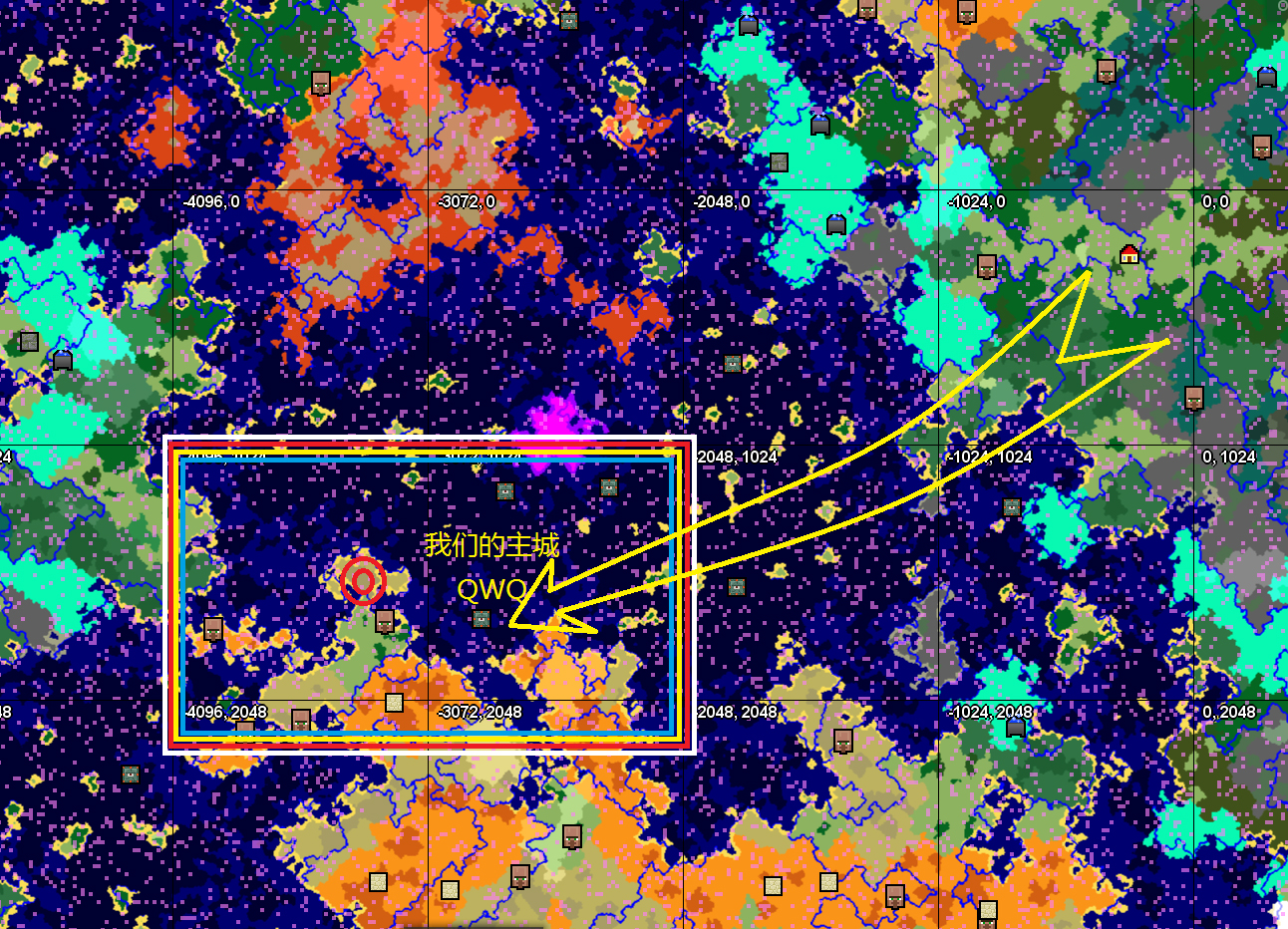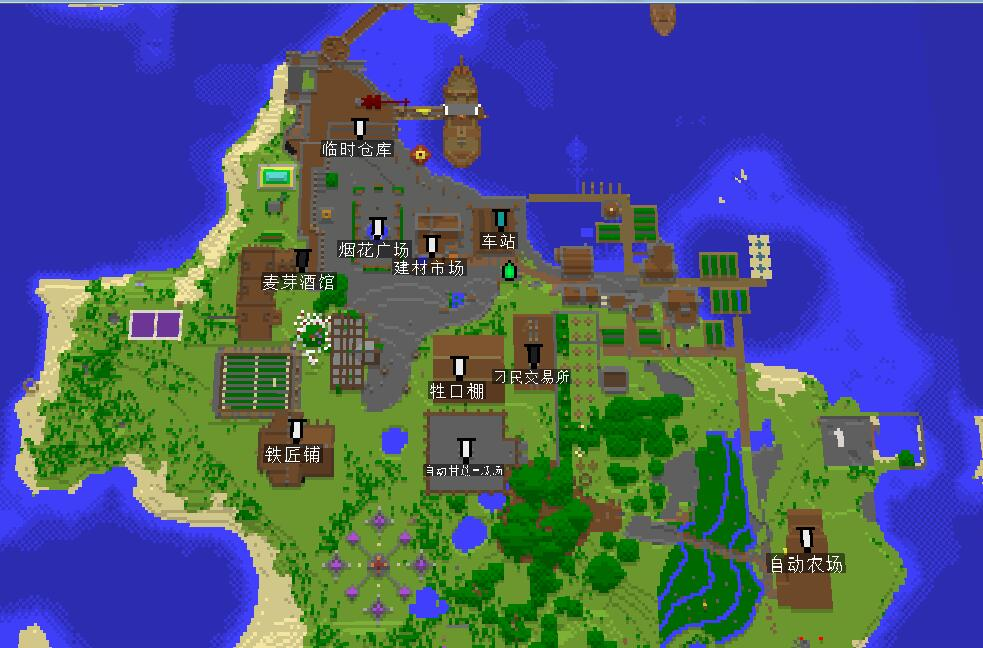最新话题

# 【西盟半岛】申请贴——生存大佬之间苟活的建筑玩家有多么不容易QAQ

• ``````* 【源起】
公元二〇一八年末月七日夜间，Vestin、ImShiKaRi两人来到这片土地，在这里踏出了方块人史上的第一步......
``````
``````=+=+=+=+=+=+=+=+=+=+=+=+=+=+=+=+=+=+=+=+=+=+=+=+=+=+=+=+=+=+=+=+=+=+=+=+=+=+=+=+=+=+=+=+=+=+=+=+=+=+=+=+=+=+=+=+=+=+=+=+=+=+=+=+=+=+=+=+=+=+=+=
``````
• 简介①_西盟半岛隶属于TIS外服，致力于建成TIS外服大陆的西方驿站，我们的目标就是TIS外服的西道主！（坚定.jpg）
②_我们将建筑作为主导发展方向，至此热切欢迎各位建筑大佬及萌新加入我们！
③_当然，生存大佬同样欢迎~ 但主岛生存器械必须整合进建筑物，效率放在后位，好看才是本体QWQ。
④_作为主岛，我们以旧世纪北欧风、中世纪魔幻风、蒸汽朋克风及工业风作为主要建筑风格。
⑤_不欢迎：有事没事拿着打火石/喜欢TNT硬核垫脚/好奇三叉戟在雷雨天的新特性/测试鼠标左键。以上。
``````=+=+=+=+=+=+=+=+=+=+=+=+=+=+=+=+=+=+=+=+=+=+=+=+=+=+=+=+=+=+=+=+=+=+=+=+=+=+=+=+=+=+=+=+=+=+=+=+=+=+=+=+=+=+=+=+=+=+=+=+=+=+=+=+=+=+=+=+=+=+=+=
``````
• 关于选址（坐标‘-3326，1650）``````=+=+=+=+=+=+=+=+=+=+=+=+=+=+=+=+=+=+=+=+=+=+=+=+=+=+=+=+=+=+=+=+=+=+=+=+=+=+=+=+=+=+=+=+=+=+=+=+=+=+=+=+=+=+=+=+=+=+=+=+=+=+=+=+=+=+=+=+=+=+=+=
``````
• 主岛发展（上帝视图）``````=+=+=+=+=+=+=+=+=+=+=+=+=+=+=+=+=+=+=+=+=+=+=+=+=+=+=+=+=+=+=+=+=+=+=+=+=+=+=+=+=+=+=+=+=+=+=+=+=+=+=+=+=+=+=+=+=+=+=+=+=+=+=+=+=+=+=+=+=+=+=+=
``````
• 申请加入方式[ ----------- 回复内容 ----------- ]
称呼(惯用名)：
游戏ID：
QQ：
[ ------------------------------------ ]
*我们（误，就岛主在）会不定时查看回复并给予企鹅群号码。
*进群请第一时间查看群置顶公告，填写成员统计表格并艾特群主协助添加白名单。
``````=+=+=+=+=+=+=+=+=+=+=+=+=+=+=+=+=+=+=+=+=+=+=+=+=+=+=+=+=+=+=+=+=+=+=+=+=+=+=+=+=+=+=+=+=+=+=+=+=+=+=+=+=+=+=+=+=+=+=+=+=+=+=+=+=+=+=+=+=+=+=+=
``````以上

• 称呼(惯用名)：阿卡
QQ：1203274984

• 称呼：Zwei
游戏ID：ZweiTriy
QQ:1395115065
擅长：除了生存啥都行

• 你们这是占了一整个岛吗hhhh

• 称呼(惯用名)：NewBeeFly
游戏ID：[email protected]
QQ：877250689

• 称呼:小梦
QQ：945776293
ID:Yuan_Meng233
萌新，对建筑方面有着非常大的爱好，生存强，希望在服务器里多多交朋友，跟大佬一起玩~

• 称呼(惯用名)：蓝球
游戏ID：Dai_Loh
QQ：983745965
萌新，建筑一般，所以想进来学习学习。

• QQ;1440334143
在线时间：每天只要不上课几乎都在线
ID:SoraAsuga
MC6年老玩家吧，算是会一些建筑之前过了国建2审（大概在15年），比较喜欢做多人大型生存建筑

• 称呼（惯用名）：药糖
游戏ID: Yao_Tang
QQ: 3072759992

• 称呼(惯用名)：嘿咻233
游戏ID：heixiu233
QQ：841975047
大学生一枚，时间还算充裕，第一次接触在13年，在旧版有稍微研究过红石，喜欢团队合作类，能肝

• 惯用名：小城
游戏ID：qYoAoYp
qq：2453991849
红石玩的不太明白，但是建筑生存还是能的，主要是喜欢多人游戏，能一起玩很棒。

• 惯用名:usb 游戏id:rundo53 擅长:生存，业余:建筑
红 qq3253089506

• 称呼(惯用名)：叫我Gr就行
游戏ID：GriRet
QQ：1677343686
玩了至少7年的mc，基本没断，实时追踪新版本更新计划，十分清楚mc的游玩与基础特性，热爱与他人一起合作，建设。
我是一个玩红石的，红石现在卡在模电这个瓶颈，模电之下基本都会，除了bud较为不熟。希望在聚落里找到志趣相合的玩家，并且提升自己的红石技术，我非常爱学新东西。

• 谢谢大佬们粗体字

• 称呼(惯用名)：叫我Gr就行
游戏ID：GriRet
QQ：1677343686
玩了至少7年的mc，基本没断，实时追踪新版本更新计划，十分清楚mc的游玩与基础特性，热爱与他人一起合作，建设。
我是一个玩红石的，红石现在卡在模电这个瓶颈，模电之下基本都会，除了bud较为不熟。希望在聚落里找到志趣相合的玩家，并且提升自己的红石技术，我非常爱学新东西。

• emmmm,打扰了，管理要是看到我的帖子请帮我清掉吧，我已经在申请鸽子镇了

• 称呼(惯用名)：甲鱼
游戏ID：destiny_wenyi
QQ：2729816872

• 称呼（惯用名）：JHIN
游戏id：JHIN537
QQ：1822605932

• ID:looook4
QQ:1203822679
极限生存党，低级红石，中级建筑.

• @Vestin 惯用名:zyl
ID:a2240692661
QQ:2240692661